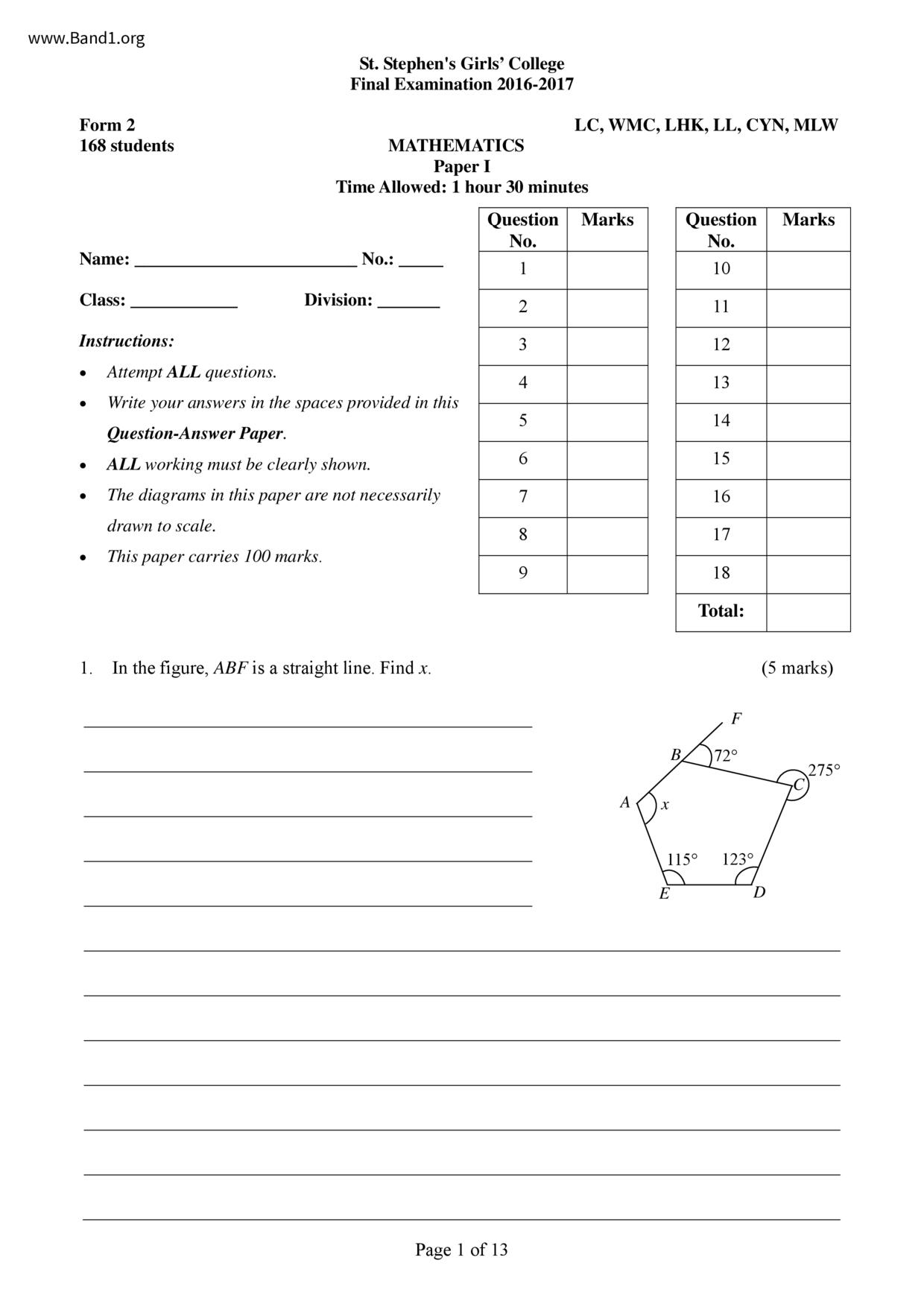# 中二 數學試卷 (F2 Maths Past Paper)

6949

pdf

13

st_stephen_girl_college_F2-Maths-P1-16-17-fy

▼ 圖片只作預覽, 如欲下載整份卷, 請按「免費成為會員」 ▼▲ 圖片只作預覽, 如欲下載整份卷, 請按「免費成為會員」 ▲

## 中二數學試卷 PDF 下載

168 students
Instructions:
St. Stephen's Girls' College
Final Examination 2016-2017
MATHEMATICS
Time Allowed: 1 hour 30 minutes
Attempt ALL questions.
ALL working must be clearly shown.
The diagrams in this paper are not necessarily
drawn to scale.
This paper carries 100 marks.
In the figure, ABF is a straight line. Find x.
Question Marks
Page 1 of 13
LC, WMC, LHK, LL, CYN, MLW
Question Marks
115° 123°
F.2 Mathematics Paper I
Final Examination 2016-2017
14. In the figure, when sun rays make an angle of 18° with the horizontal ground, AQ is the shadow
of a vertical pole PQ. It is known that when sun rays make an angle of 25° with the horizontal
ground, the shadow of the pole becomes BQ which is 0.5 m shorter than AQ. Suppose BQ is x m.
(a) By considering APBQ, express PQ in terms of x.
(b) By considering APAQ, express PQ in terms of x.
(c) Hence, find the height of the pole PQ. (Correct your answer to 3 significant figures.)
Page 10 of 13
F.2 Mathematics Paper I
15. (a) Factorize 8c + 24.
(b) Factorize ac + bc + 3a + 3b.
(c) Using the results of (a) and (b), factorize ac + bc + 3a + 3b + 8c +24.
(b) Make a the subject of the following formula, where a + b +0:
16. (a) Simplify +
Final Examination 2016-2017
b a b-1 a +1
Page 11 of 13
F.2 Mathematics Paper I
17. (a) Prove that (a + b)²(a − b)² = (a² − b²)².
(b) Expand (a²-6²)².
(c) It is known that a² + b² = 10 and ab = 3.
(i) Find the value of (a + b)².
(ii) Hence, or otherwise, find the value of a4 - 2a²b² + b4.
Final Examination 2016-2017
Page 12 of 13
F.2 Mathematics Paper I
18. (a) Expand and simplify (sin x + cos x)².
(b) Expand and simplify (sin x - cos x)².
Final Examination 2016-2017
(c) Given that 45° (a) and (b).
End of Paper
Page 13 of 13
F.2 Mathematics Paper I
Final Examination 2016-2017
2. In the figure, AD and BC intersect at E and AD BC. It is given that AC = DC, AB // CD and
(a) Find ZBCD.
3. The given figure is formed by five straight lines. Find a +b+c+d+e.
Page 2 of 13
F.2 Mathematics Paper I
Final Examination 2016-2017
4. In the figure, ABCD is a rectangle. If E is a point lying on AD such that ZABE = 50°, find ZCED.
Page 3 of 13
5. In the figure, AB = AE, ZBCD= ZEDC = 90° and ZBAE = 140°. If BC = CD=DE = 8 cm, find
the perimeter of the pentagon ABCDE. (Correct your answer to 3 significant figures.) (4 marks)
F.2 Mathematics Paper I
6. (a) Complete the table for the equation y = -2x + 3.
Final Examination 2016-2017
(b) According to the table in (a), draw the graph of y=-2x +3 on the graph paper provided
Page 4 of 13
F.2 Mathematics Paper I
Final Examination 2016-2017
7. The histogram below shows the distribution of the time that a group of ladies spend on doing
exercise every day.
Time that a group of ladies spend
on doing exercise every day
29.5 59.5 89.5 119.5 149.5
(a) Complete the following frequency distribution table according to this histogram. (4 marks)
Class Boundaries (min)
Class Mark (min)
(b) Complete the corresponding cumulative frequency distribution table.
Time less than (min) 29.5
Cumulative frequency
(c) What percentage of ladies spend 89.5 min or more on doing exercise every day? (Correct
Page 5 of 13
F.2 Mathematics Paper I
8. If (2a + b) (3a + 4b) = 2:5, find a : b.
9. (a) Rationalize the denominator of
(b) Hence, simplify +3√5.
Final Examination 2016-2017
Page 6 of 13
F.2 Mathematics Paper I
10. It is given that a = √5 and b=√7. Express √√35+√140 in terms of a and b.
Final Examination 2016-2017
11. (a) Simplify 2 sin x + cos x tan x.
(b) Without using a calculator, find the value of
Page 7 of 13
in surd form and rationalize the
F.2 Mathematics Paper I
Final Examination 2016-2017
12. A building is 44 cm high in a photograph. It is known that the actual height of the building is
96.8 m and the actual width of the building is 15.4 m.
(a) Find the scale of the photograph in the form 1: n.
(b) Find the width (in cm) of the building in the photograph.
Page 8 of 13
F.2 Mathematics Paper I
Final Examination 2016-2017
13. In the figure, C is a point on BD. AD and EC intersect at F. ZBDE = 90°, AB = 48, BD = 55,
AD = 73, CE = 125 and BC: CD = 1:4.
(a) Find the length of DE.
(b) Prove that ZABD = 90°.
(c) Find the area of the quadrilateral ABDE.
Page 9 of 13